The COSAN Model

The original COSAN (covariance structure analysis) model is proposed by McDonald (1978, 1980) for analyzing general covariance structure models. PROC CALIS enables you to analyze a generalized form of the original COSAN model. The generalized COSAN model extends the original COSAN model with the inclusion of addition terms in the covariance structure formula and the associated mean structure formula.

The covariance structure formula of the generalized COSAN model is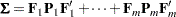and the corresponding mean structure formula of the generalized COSAN model is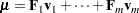where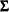is a symmetric correlation or covariance matrix for the observed variables,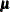is a vector for the observed variable means, each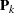is a symmetric matrix, each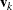is a mean vector, and each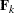(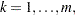) is the product of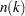matrices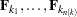; that is,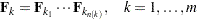The matrices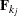andin the model can be one of the forms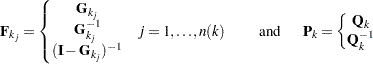where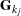and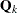are basic model matrices that are not expressed as functions of other matrices.

The COSAN model matrices and vectors are,, and(when the mean structures are analyzed). The elements of these model matrices and vectors are either parameters (free or constrained) or fixed values. Matrixis referred to as the central covariance matrix for theth term in the covariance structure formula.

Essentially, the COSAN modeling language enables you to define the covariance and mean structure formulas of the generalized COSAN model, the basic COSAN model matrices,, and, and the parameters and fixed values in the model matrices.

You can also specify a generalized COSAN model without using an explicit central covariance matrix in any term. For example, you can define theth term in the covariance structure formula as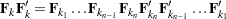The corresponding term for the mean structure becomes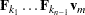In the covariance structure formula,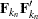serves as an implicit central covariance matrix in this term of the covariance structure formula. Because of this,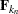does not appear in the corresponding mean structure formula.

To take advantage of the modeling flexibility of the COSAN model specifications, you are required to provide the correct covariance and mean structure formulas for the analysis problem. If you are not familiar with the mathematical formulations of structural equation models, you can consider using simpler modeling languages such as PATH or LINEQS.

### An Example: Specifying a Second-Order Factor Model

This example illustrates how to specify the covariance structures in the COSAN statement. Consider a second-order factor analysis model with the following formula for the covariance structures of observed variables v1-v9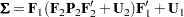where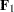is a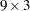first-order factor matrix,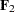is a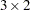second-order factor matrix,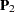is a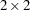covariance matrix for the second-order factors,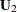is a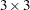diagonal matrix for the unique variances of the first-order factors, and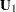is a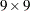diagonal matrix for the unique variances of the observed variables.

To fit this covariance structure model, you first rewrite the covariance structure formula in the form of the generalized COSAN model as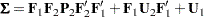You can specify the list of observed variables and the three terms for the covariance structure formula in the following COSAN statement:

```cosan var= v1-v9,
F1(3) * F2(2) * P2(2,SYM) + F1(3) * U2(3,DIA) + U1(9,DIA);
```

The VAR= option specifies the nine observed variables in the model. Next, the three terms of the covariance structure formula are specified. Because each term in the covariance structure formula is a symmetric product, you only need to specify each term up to the central covariance matrix. For example, although the first term in the covariance structure formula is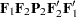, you only need to specify F1(3) * F2(2) * P2(2,SYM). PROC CALIS generates the redundant information for the term. Similarly, you specify the other two terms of the covariance structure formula.

In each matrix specification of the COSAN statement, you can specify the following three matrix properties as the arguments in the trailing parentheses: the number of columns, the matrix type, and the transformation of the matrix. For example, F1(3) means that the number of columns of F1 is(while the number of rows isbecause this number has to match the number of observed variables specified in the VAR= option), F2(2) means that the number of columns of F2 is(while the number of rows isbecause the number has to match the number of columns of the preceding matrix, F1). You can specify the type of the matrix in the second argument. For example, P2(2,SYM) means that P2 is a symmetric (SYM) matrix and U2(2,DIA) means that U2 is a diagonal (DIA) matrix. You can also specify the transformation of the matrix in the third argument. Because there is no transformation needed in the current second-order factor model, this argument is omitted in the specification. See the COSAN statement for details about the matrix types and transformation that are supported by the COSAN modeling language.

Suppose now you also want to analyze the mean structures of the second-order factor model. The corresponding mean structure formula is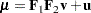whereis a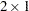mean vector for the second-order factors andis a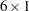vector for the intercepts of the observed variables. To analyze the mean and covariance structures simultaneously, you can use the following COSAN statement:

```cosan var= v1-v9,
F1(3) * F2(2) * P2(2,SYM) [mean = v] + F1(3) * U2(3,DIA)
+ U1(9,DIA) [mean = u];
```

In addition to the covariance structure specified, you now add the trailing MEAN= options in the first and the third terms. PROC CALIS then generates the mean structure formula by the following steps:

• Remove the last matrix (that is, the central covariance matrix) in each term of the covariance structure formula.

• Append to each term the vector that is specified in the MEAN= option of the term, or if no MEAN= option is specified in a term, that term becomes a zero vector in the mean structure formula.

Following these steps, the mean structure formula generated for the second-order factor model is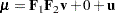which is what you expect for the mean structures of the second-order factor model. To complete the COSAN model specification, you can use MATRIX statements to specify the parameters and fixed values in the COSAN model matrices. See Example 26.28 for a complete example.

### Special Cases of the Generalized COSAN Model

It is illustrative to see how you can view different types of models as a special case of the generalized COSAN model. This section describes two such special cases.

#### The Original COSAN Model

The original COSAN (covariance structure analysis) model (McDonald; 1978, 1980) specifies the following covariance structures: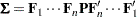This is the generalized COSAN with only one term for the covariance structure model formula. Hence, using the COSAN statement to specify the original COSAN model is straightforward.

#### Reticular Action Model

The RAM (McArdle; 1980; McArdle and McDonald; 1984) model fits the covariance structures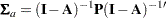where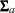is the symmetric covariance for all latent and observed variables in the RAM model,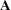is a square matrix for path coefficients,is an identity matrix with the same dimensions as, and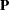is a symmetric covariance matrix. For details about the RAM model, see the section The RAM Model.

Correspondingly, the RAM model fits the mean structure formula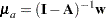where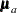is the mean vector for all latent and observed variables in the RAM model andis a vector for mean or intercepts of the variables.

To extract the covariance and mean structures for the observed variables, a selection matrix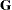is used. The selection matrixcontains zeros and ones as its elements. Each row ofhas exactly one nonzero element at the position that corresponds to the location of a manifest row variable inor. The covariance structure formula for the observed variables in the RAM model becomes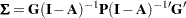The mean structure formula for the observed variables in the RAM model becomes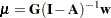These formulas suggest that the RAM model is special case of the generalized COSAN model with one term. For example, suppose that there are
observed variables (var1var10) andlatent variables in a RAM model. The following COSAN statement represents the RAM model:

```cosan var= v1-v10,
G(13,GEN) * A(13,GEN,IMI) * P(13,SYM) [Mean = w];
```

In the COSAN statement, you define the 10 variables in the VAR= option. Next, you provide the formulas for the mean and covariance structures.is
general matrix (GEN),is a
general matrix with the IMI transformation (that is,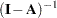),is asymmetric matrix (SYM), andis a
vector. With these COSAN statement specifications, your mean and covariance structure formulas represent exactly those of the RAM model. To complete the entire model specification, your next step is to use the MATRIX statements to specify the parameters and fixed values in the model matrices,,, and.

Similarly, it is possible to use the COSAN modeling language to represent any other model types such as models defined by the FACTOR, LINEQS, LISMOD, MSTRUCT, PATH, and RAM statements. But this is not an automatic recommendation of using the COSAN modeling languages in all situations. When an analysis can be specified by either the COSAN or a more specific modeling language (for example, PATH), you should consider using the specific modeling language because the specific modeling language can exploit specific model features so that it does the following:

• enables more supplemental analysis (effect analysis, standardized solutions, and so on), which COSAN has no general way to display

• supports better initial estimation methods (the COSAN model can only set initial estimates to certain default or random values)

• leads to more efficient computations due to the availability of more specific formulas and algorithms

Certainly, the COSAN modeling language is still very useful when you fit some nonstandard model structures that cannot be handled otherwise by the more specific modeling languages.

### Naming Variables in the COSAN Model

Although you can define the list of observed (manifest) variables in the VAR= option of the COSAN statement, the COSAN modeling language does not support a direct specification of the latent or error variables in the model. In the COSAN statement, you can define the model matrices and how they multiply together to form the covariance and mean structures. However, except for the row variables of the first matrix in each term, you do not need to identify the row and column variables in all other matrices. However, you can use the VARNAMES statement to label the column variables of the matrices. The names in the VARNAMES statement follow the general naming rules required by the general SAS system. They should not contain special characters and cannot be longer than 32 characters. Also, they do not need to use certain prefixes like what the LINEQS modeling language requires. It is important to realize that the VARNAME statement only labels, but does not identify, the column variables (and the row variables, by propagation). This means that while keeping all other things equal, changing the names in the VARNAMES statements does not change the mathematical model or the estimation of the model. For example, you can label all columns of a COSAN matrix with the same name but it does not mean that these columns refer to the same variable in the model. See the section Naming Variables and Parameters for the general rules about naming variables and parameters.

### Default Parameters in the COSAN Model

The default parameters of the COSAN model matrices depend on the types of the matrices. Each element of the IDE or ZID matrix (identity matrix with or without an additional zero matrix) is either a fixed one or a fixed zero. You cannot override the default parameter values of these fixed matrices. For COSAN model matrices with types other than IDE or ZID, all elements are fixed zeros by default. You can override these default zeros by specifying them explicitly in the MATRIX statements.